Instant Maths Help:

# Edexcel A Level

## Differentiation from First Principles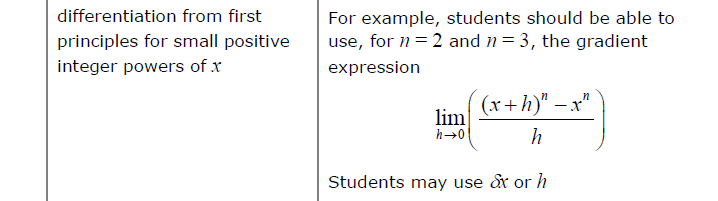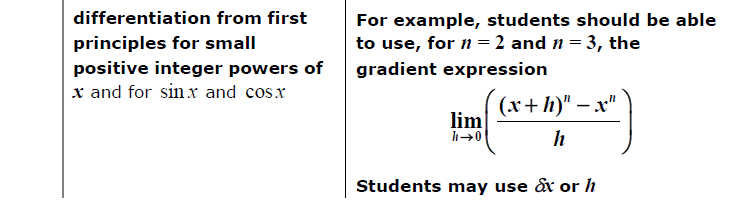##### Edexcel 9MA0/01 Jun 2023 A2 Exam Q. 12 :   5 marks in 6:00 min.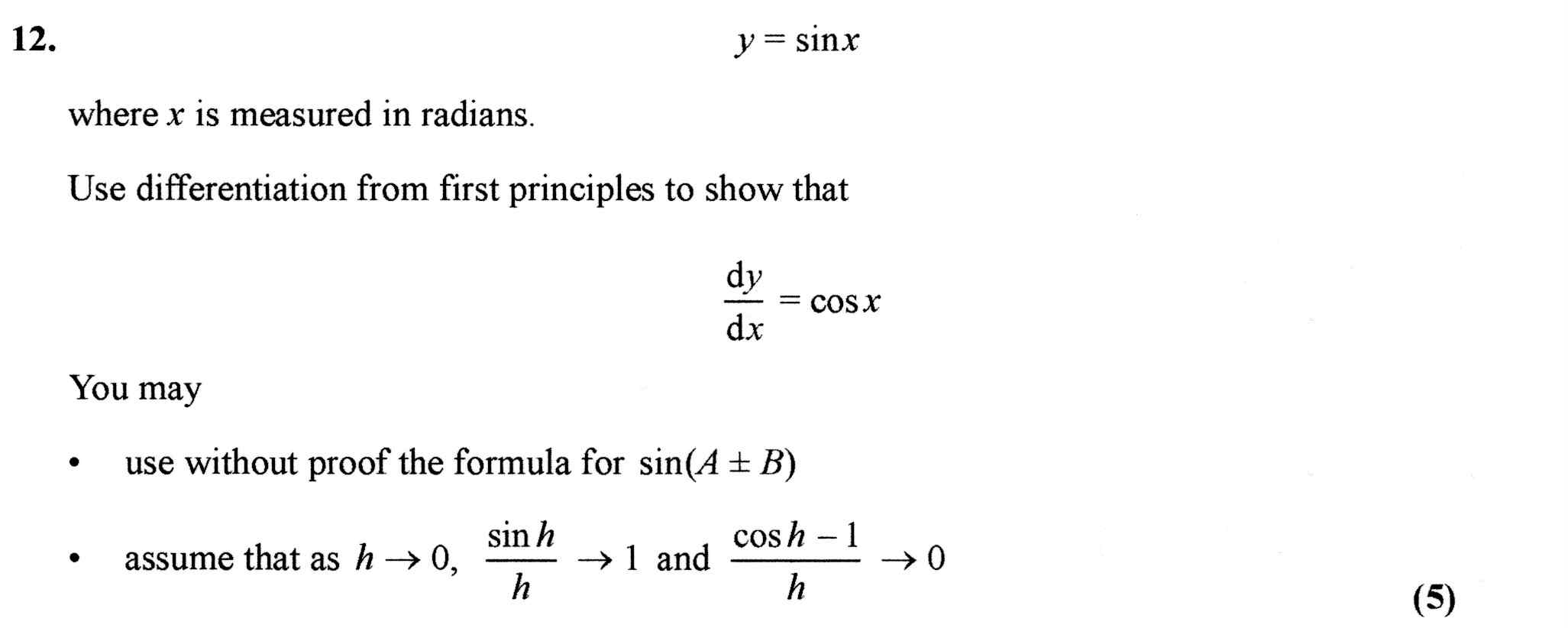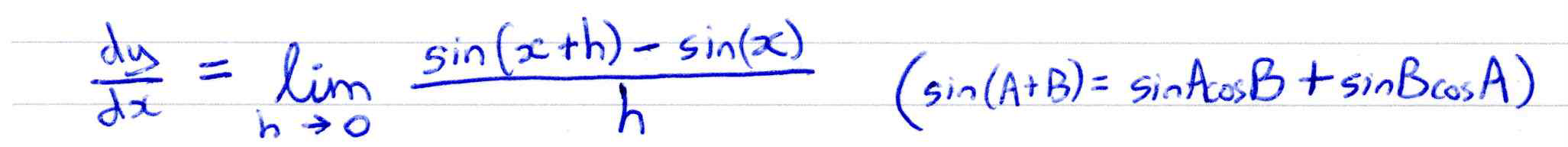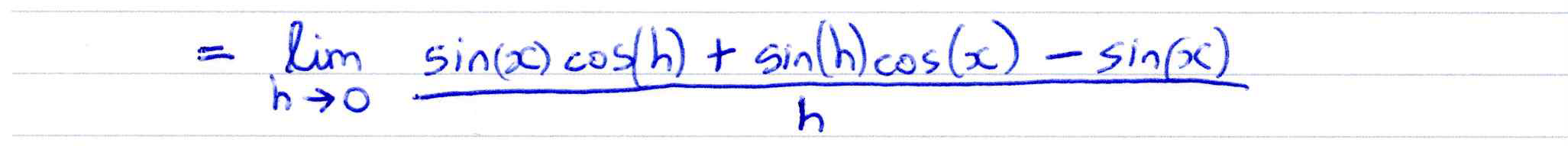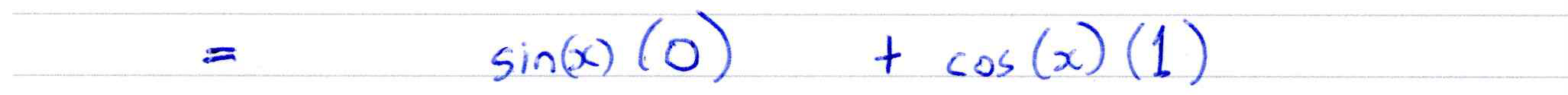##### Derivative as limit of chord gradient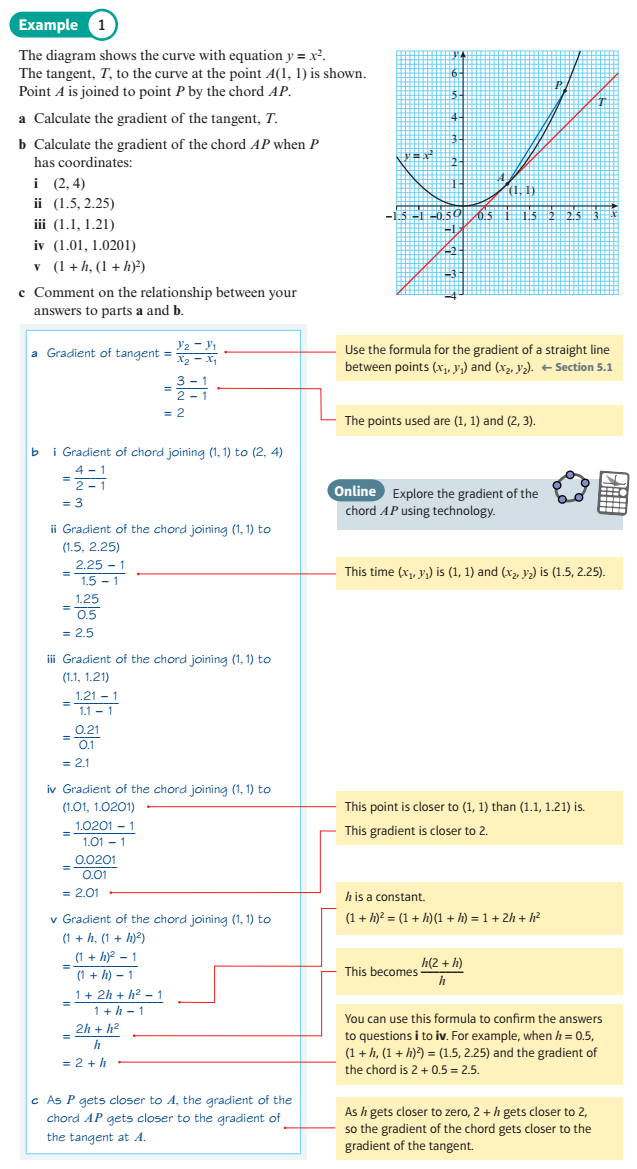##### Chords and Tangents of Polynomials
You can use this display to see how the gradient of a chord (or secant) approaches the gradient of a tangent as the length of the chord approaches zero.

Having defined your polynomial, with the blue sliders, you can set the tangent point with the "tangent x" slider, and then drag an approaching point towards it with the "approach x" slider.
##### Chords and Tangents of Trig Functions
You can use this display to see how the gradient of a chord (or secant) approaches the gradient of a tangent as the length of the chord approaches zero.

Having selected your trigonometric function, you can set the tangent point with the "tangent x" slider,